# Product (mathematics) facts for kids

Kids Encyclopedia Facts

In mathematics, a product is a number or a quantity obtained by multiplying two or more numbers together. For example: 4 × 7 = 28 Here, the number 28 is called the product of 4 and 7. The product of 6 and 4 will be 24, because 6 × 4 = 24.

## Capital pi

A short notation for long multiplication expressions is the product notation. It uses the capital Greek letter pi: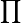$\prod$. This works the same as the Sigma notation. Informally, given a sequence of numbers (or elements of a multiplicative structure with unit) say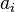$a_i$ we define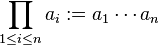$\prod_{1\leq i\leq n}a_i:=a_1\dotsm a_n$. A rigorous definition is usually given recursively as follows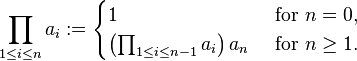$\prod_{1\leq i\leq n}a_i := \begin{cases} 1 & \text{ for } n=0 , \\ \left(\prod_{1\leq i\leq n-1}a_i\right) a_n & \text{ for } n\geq1 . \end{cases}$

An alternative notation for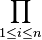$\prod_{1\leq i\leq n}$ is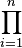$\prod_{i=1}^n$.

### Properties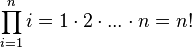$\prod_{i=1}^n i = 1 \cdot 2 \cdot ... \cdot n = n!$ (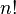$n!$ is pronounced "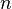$n$ factorial" or "factorial of$n$");$\prod_{i=1}^n x = x^n$, i.e., the usual$n$th power operation;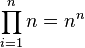$\prod_{i=1}^n n = n^n$, i.e., we multiply$n$ by itself$n$ times;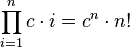$\prod_{i=1}^n c \cdot i = c^n \cdot n!$ where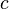$c$ is a constant with respect to$i$.

From the above equation we can see that any number with an exponent can be represented by a product, though it normally is not desirable.

Unlike summation, the sums of two terms cannot be separated into different sums. That is,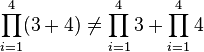$\prod_{i=1}^4 (3 + 4) \neq \prod_{i=1}^4 3 + \prod_{i=1}^4 4$,

This can be thought of in terms of polynomials: one generally cannot separate terms inside them before they are raised to an exponent. But the product does,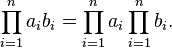$\prod_{i=1}^n a_ib_i=\prod_{i=1}^na_i\prod_{i=1}^nb_i.$

### Relation to Summation

The product of powers with the same base can be written as an exponential of the sum of the powers' exponents: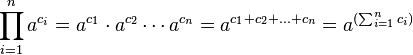$\prod_{i=1}^n a^{c_i} = a^{c_1} \cdot a^{c_2} \dotsm a^{c_n}= a^{c_1 + c_2 + ... + c_n} = a^{(\sum_{i=1}^n c_i)}$

## Images for kidsProduct (mathematics) Facts for Kids. Kiddle Encyclopedia.